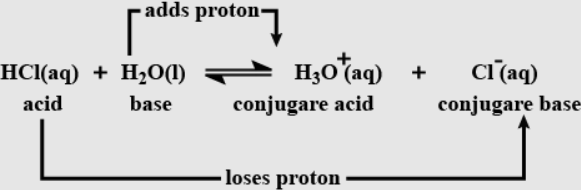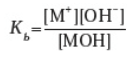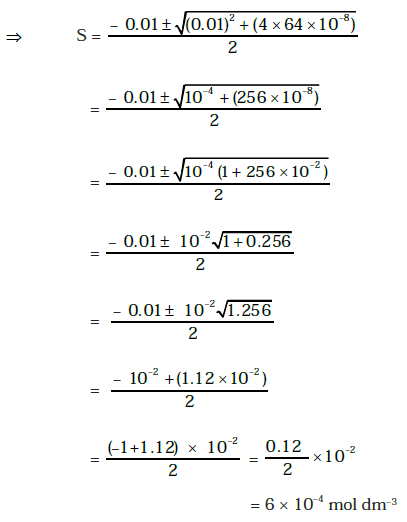Checkout JEE MAINS 2022 Question Paper Analysis : Checkout JEE MAINS 2022 Question Paper Analysis :

# Important Questions for Class 11 Chemistry Chapter 7 - Equilibrium.

Class 11 chemistry important questions with answers are provided here for Chapter 7 – Equilibrium. These important questions are based on the CBSE board curriculum and correspond to the most recent Class 11 chemistry syllabus. By practising these Class 11 important questions, students will be able to quickly review all of the ideas covered in the chapter and prepare for the Class 11 Annual examinations as well as other entrance exams such as NEET and JEE.

Download Class 11 Chemistry Chapter 7 – Equilibrium Important Questions with Answers PDF by clicking on the button below.

## Class 11 Equilibrium Important Questions with Answers

Q1. The ionisation of hydrochloric in water is given below:

HCI(aq) + H2O (l) ⇋ H3O+ (aq) + CI (aq)

Label two conjugate acid-base pairs in this ionisation.

Conjugate acid and conjugate base are marked as follows.Q2. The aqueous solution of sugar does not conduct electricity. However, when sodium

chloride is added to water, it conducts electricity. How will you explain this statement on

the basis of ionisation and how is it affected by the concentration of sodium chloride?

Sugar is a non-electrolyte, and when it dissolves in water, there will be no ionisation. If there are no free ions, it will not conduct electricity. In contrast, When sodium chloride is added to water, it is wholly ionised to give Na+ ions and Cl ions. Due to the presence of ions, there will be electrical conductance as ions are carriers of electric current.

Q3. BF3 does not have protons but still acts as an acid and reacts with NH3. Why is it so? What

type of bond is formed between the two?

BF3 is electron-deficient and hence acts as Lewis acid. : NH3 has one lone pair, which can donate to BF3 and form a coordinate bond with NH3, i.e. (NH3: → BF3).

Q4. The ionisation constant of a weak base MOH, is given by the expressionValues of the ionisation constant of some weak bases at a particular temperature are given below:

Base Dimethylamine Urea Pyridine Ammonia
Kb 5.4 x 10-4 1.3 x 10-14 1.77× 10-9 1.77 × 10-5

Arrange the bases in decreasing order of the extent of their ionisation at equilibrium. Which

of the above base is the strongest?

Higher the value of Kb, the stronger will be the base. Among the given bases, the most substantial base is Dimethylamine.

Dimethylamine > Ammonia > Pyridine > Urea.

Q5. Conjugate acid of a weak base is always stronger. The decreasing order of basic strength of the following conjugate bases will be?

OH, RO, CH3COO, CI

Conjugate acids of given bases are H2O, ROH, CH3COOH, HCl.

Their acidic strength is in the order.

HCl > CH3COOH > H2O >ROH.

Hence, their conjugate bases will have strength in the order.

RO > OH > CH3COO > CI

Q6. Arrange the following in increasing order of pH.

KNO3 (aq), CH3COONa (aq), NH4Cl (aq), C6H5COONH4 (aq)

NH4Cl < C6H5COONH4 < KNO3 < CH3COONa

KNO3 is formed from a strong acid, nitric acid and strong base, potassium hydroxide. Salts of strong acid and strong base hydrolyse to form a neutral solution. Thus its pH will be nearly 7. Sodium acetate is formed from a weak acid, acetic acid, and strong base sodium hydroxide. Thus its pH will be more than 7.C6H5COONH4 is formed from a mild acid, C6H5COOH and a weak base, NH4OH. Thus its pH will be less than 7. NH4Cl is formed from a weak base, NH4OH, and strong acid, HCl. Thus its pH value would be less than 5.

Q7. The value of Kc for the reaction 2HI (g) ⇋ H2 (g) + I2 (g) is 1 x 10-4. At a given time, the composition of reaction mixture is [HI] = 2 x 10-5 mol, [I2 ] = 1 x 10-5 mol. Determine the direction in which the reaction will proceed?

At a given time, the reaction quotient Q for the reaction will be given by the expression.

Q = [H2 ][I2 ] / [HI]

Q = 1 X 10-5 X 1 X 10-5 / (2 X 10-5 )2

Q = 1 / 4

Q = 0.25

Q = 2.5 X 10-1

As the value of the reaction quotient is greater than the value of Kc , i.e. 1×10−4, the reaction will proceed in the reverse direction.

Q8. On the basis of the equation pH = – log [H+], the pH of 10-8 mol dm-3 solution of HCI

should be 8. However, it is observed to be less than 7.0. Explain the reason.

Here, the water concentration cannot be neglected since the solution is very dilute.

[H3O+] = 10−8 +10−7 M

[H3O+] = 10−8 (1 + 10)

[H3O+] = 11 X 10−8 M

pH = – log [H3O+]

pH = – log 11 X 10−8 M

pH = 8 – log 11

pH = 8 – 1.04

pH = 6.96

pH will be less than 7.0.

Q9. The pH of a solution of a strong acid is 5.0. What will be the pH of the solution obtained after diluting the given solution 100 times?

pH = 5 means [H+] = 10−5

On diluting 100 times ,

[H+] = 10−5 / 100 = 10−7

On calculating the pH using the equation pH = − log [H+], the pH value comes out to be 7, which is impossible.

Hence, Total H+ ion concentration = H+ ions from acid + H+ ion from water

[H+] = 10−7 +10−7 M

[H+] = 2 X 10−7

pH = 7 – 0.3010

pH = 6.699

Q10. A sparingly soluble salt gets precipitated only when the product of the concentration of its ions in the solution (Qsp) becomes greater than its solubility product. If the solubility of BaSO4

in water is 8 × 10-4 mol dm-3. Calculate its solubility in 0.01 mol dm-3 of H2SO4

BaSO4 (s) ⇋ Ba 2+ (aq) + SO42- (aq)
At t = 0 1 0 0
At equilibrium in water 1 – S S S
At equilibrium in the presence of sulphuric acid 1 – S S S + 0.01

Ksp for BaSO4 in water = [ Ba 2+ ] [ SO42- ] = (S) (S) = S2

But S = 8 × 10–4 mol dm–3

∴ Ksp = (8 × 10–4 )2

= 64 × 10–8 … (1)

The expression for Ksp in the presence of sulphuric acid will be as follows.

Ksp = (S) (S + 0.01) … (2)

Since the value of Ksp will not change in the presence of sulphuric acid, therefore from (1) and (2)

(S) (S + 0.01) = 64 × 10–8

S2 + 0.01 S = 64 × 10–8

S2 + 0.01 S – 64 × 10–8 = 0Q11. The pH of 0.08 mol dm-3 HOCI solution is 2.85. Calculate its ionisation constant.

pH of HOCl = 2.85

But, – pH = log [H+]

∴ -2.85 = log [H+]

-3.15 = log [H+] [H+] = 1.413 × 10–3

For the weak monobasic acid [H+] = (Ka X C) 1 / 2

Ka = [H+] 2 / C

Ka = (1.413 × 10–3 )2 / 0.08

Ka = 24.957 × 10–6

Ka = 2.4957 × 10–5

Q12. Calculate the pH of a solution formed by mixing equal volumes of two solutions A and B of

a strong acid having pH = 6 and pH = 4 respectively.

The pH of solution A = 6,

Therefore, the concentration of [H+] ion in solution A=10−6 mol / L

The pH of solution B = 4

Therefore, the concentration of [H+] ion in solution B=10−4mol / L.

On mixing one litre of each solution, total volume = 1L + 1L = 2L.

Amount of H+ ions in 1L of solution A = concentration × Volume (V) =

Amount of H+ ions in 1L of solution A = 10−6 X 1 = 10−6

Amount of H+ ions in 1L of solution B =10−4 X 1 = 10−4

∴ The total amount of H+ ions in the solution formed by mixing solutions A and B is (10−5 mol + 10−4 mol)

This amount is present in 2L solution,

∴ Total [H+] = 10−4 (1 + 1.01) / 2 mol / L

Total [H+] = 1.01 X 10−4 / 2 mol / L

Total [H+] = 0.5 X 10−4 mol / L

Total [H+] = 5 X 10−5 mol / L

pH = – log [H+]

pH = – log [5 X 10−5]

pH = – [ log 5 – 5 log 10]

pH = – log 5 + 5

pH = 5 – log 5

pH = 5 – 0.6990

pH = 4.3010

pH = 4.3

Thus, the pH will be 4.3.

Q13. The solubility product of Al(OH)3 is 2.7 x 10-11. Calculate its solubility in g / L and also find out the pH of this solution. (Atomic mass of Al = 27 u).

Let S be the solubility of Al(OH)3

Ksp = [Al3+] [OH]3

Ksp = (S) (3S)3

Ksp = 27S4

S4 = Ksp / 27

S4 = 27 × 10-11 / 27 x 10

S4 = 1 x 10 -12 mol / L.

S = 1 x 10 -3 mol / L.

(i) Solubility of Al(OH)3: Molar mass of Al(OH)3 is 78g.

Therefore, the solubility of Al(OH)3 in g / L = 1×10 3 × 78 g / L

Solubility of Al(OH)3 in g / L = 78 × 10−3 g / L

Solubility of Al(OH)3 in g / L = 7.8 × 10−2 g / L

(ii) pH of the solution: S = 1 × 10−3 mol / L

[OH] = 3S = 3 × 1 × 10−3 = 3 × 10−3

pOH =3 − log 3

pH = 14 − pOH = 11 + log 3 = 11.4771.

Q14. Calculate the volume of water required to dissolve 0.1 g lead (II) chloride to get a

saturated solution. (Ksp of PbCl2 = 3.2 x 10-8, atomic mass of Pb= 207 u).

Ksp of PbCl2 = 3.2 × 10–8

Let S be the solubility of PbCl2.

PbCl2 (s) ⇋ Pb 2+ (aq) + 2 Cl (aq)
Concentration of specie at t = 0 1 0 0
Concentration of various specie at equilibrium 1 – S S 2S

Ksp = [ Pb 2+][Cl ]2

Ksp = (S) (2S)2

Ksp = 4S3

S3 = Ksp / 4

S3 = 3.2 x 10-8 / 4 mol / L

S3 = 8 x 10-9 mol / L

S = 2 x 10-3 mol / L

Molar Mass of PbCl2 = 278

Hence, solubilty of PbCl2 in g / L = 2 x 10-3 x 278 g / L

Solubilty of PbCl2 in g / L = 556 x 10-3 g / L

Solubilty of PbCl2 in g / L = 0.556 g / L

To get saturated solution, 0.556 g of PbCl2 is dissolved in 1 L water.

0.1g PbCl2 is dissolved in 0.1 / 0.556 L = 0.1798 L water.

To make a saturated solution, dissolution of 0.1 g PbCl2 in 0.1798 L ≈ 0.2 L of water will be required.

Q15. A reaction between ammonia and boron trifluoride is given below:

: NH3 + BF3 → H3N: BF3

Identify the acid and base in this reaction. Which theory explains it? What is the

hybridisation of B and N in the reactants?

NH3 is Lewis base, while BF3 is Lewis acid. Lewis’s electronic theory of acids and bases explains it.

The hybridisation state of nitrogen in NH3 is sp3 hybridised, and boron in BF3 is sp2 hybridised.

Q16. Following data is given for the reaction: CaCO3(s) → CaO (s) + CO2 (g)

Δf H [CaO (s)] = – 635.1 kJ / mol

Δf H [CO2 (g)] = – 393.5 kJ / mol

Δf H [CaCO3 (s)] = – 1206.9 kJ / mol

Predict the effect of temperature on the equilibrium constant of the above reaction.

Δf H = Δf H [CaO (s)] + Δf H [CO2 (g)] − Δf H [CaCO3 (s)]

∴ Δf H = 178.3 kJ / mol.

The reaction is endothermic.

Hence, according to Le Chatelier’s principle, the reaction will proceed in the forward direction on increasing temperature.

Q1. How can you predict the following stages of a reaction by comparing the value of Kc and

Qc?

(i) Net reaction proceeds in the forward direction.

(li) Net reaction proceeds in the backward direction.

(iii) No net reaction occurs.

The values of Kc and Qc are self-explanatory and less than or greater than one another decides the direction in which reaction will proceed as follows-

(i) As Qc < Kc, the reaction will proceed in the direction of products (forward direction).

(ii) If Qc > Kc, the reaction will proceed in the direction of reactants (reverse direction).

(iii) If Qc = Kc, no net reaction will occur.

Q2. On the basis of the Le Chatelier principle explain how temperature and pressure can be

adjusted to increase the yield of ammonia in the following reaction.

N2 (g) + 3H2 (g) ⇋ 2NH3 (g) ΔH = – 92.38 kJ mol-1

What will be the effect of addition of argon to the above reaction mixture at constant

volume?

According to Le Chatelier’s principle, raising the temperature shifts the equilibrium to the left and decreases the equilibrium concentration of ammonia since it is an exothermic reaction. In other words, low temperature and high pressure are favourable for a high yield of ammonia. There will be no change in equilibria on the addition of argon (Ar).

Q3. A sparingly soluble salt having general formula A P*x B9 and molar solubility S is in

equilibrium with its saturated solution. Derive a relationship between the solubility and

solubility product for such salt.

Axp+ + Byq− ⇋ xAp+ (aq) + yBq− (aq)

S moles of A B dissolve to give x S moles of Ap+ and y S moles of Bq.

Ksp = [Ap+] x [Bq−] y

Ksp =[x5] x [y5] y

x x y y 5 x+y.

Q4. Write a relation between ΔG and Q and define the meaning of each term and answer the

following:

(a) Why a reaction proceeds forward when Q < K and no net reaction occurs when Q = K.

(b) Explain the effect of an increase in pressure in terms of reaction quotient O. for the reaction: CO (g) + 3H2 (g) ⇋ CH4 (g) + H2O (g)

(a) ΔG = ΔG+ RT ln Q

ΔG = Change in free energy as the reaction proceeds,

ΔG = Standard free energy change,

Q = Reaction quotient,

R = Gas constant,

T = Absolute temperature.

Since ΔG = − RTlnK

∴ ΔG = −RTlnK + RTlnQ

ΔG = RT lnQ / K

If Q < K, ΔG will be negative. The reaction will proceed in forward direction.

If Q = K, ΔG = 0, the reaction is in equilibrium, and no net reaction is there.

(b) CO (g) + 3H2 (g) ⇋ CH4 (g) + H2O (g),

According to the Le Chatiliers principle, when the pressure increases, the equilibrium will shift in that direction where there are fewer gas molecules.

As per the available information,

The number of moles of reactants = 1 + 3 = 4

The number of moles of product = 1 + 1 = 2

Hence, the equilibrium will shift forward as the number of moles of product is less.

Test your Knowledge on Important questions class 11 chemistry chapter 7 equilibrium!